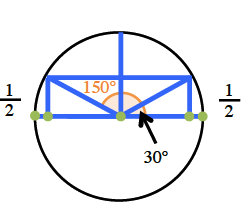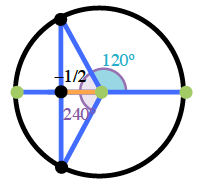### Home > PC3 > Chapter 2 > Lesson 2.3.1 > Problem2-127

2-127.

State all values of $0\leθ<2\pi$ that satisfy each of the following equations. Sketching a unit circle will help.

1. $\sin(θ)=\frac{1}{2}$$30^\circ\text{ or }\frac{\pi}{6}$

$150^\circ\text{ or }\frac{5\pi}{6}$

1. $\cos(θ)=−\frac{1}{2}$1. $\sin(θ)=−\frac{\sqrt{3}}{2}$

What special angle(s) are involved?
What does the negative sign mean?

1. $\cos(θ)=0$

These angles are coterminal with the $y$-axis.